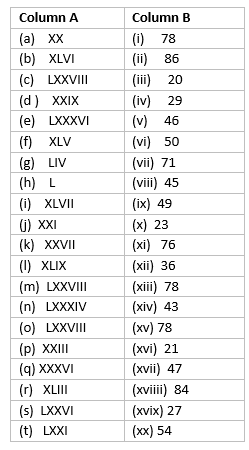# Test Paper for Class 6 Maths Chapter 1 Knowing our Numbers

In this page we have Test paper for Class 6 Maths Knowing our Numbers . If you like them and do not forget to like and share.
Question 1
Write Roman Numerals in front of each given integers
a. 46
b. 71
c. 42
d. 75
e. 68
f. 28
g. 38
h. 67
i. 64
j. 57
k. 59
l. 24
m. 44
n. 42
o. 70
p. 31
q. 62
r. 49
s. 57
t. 85

Question 2
Write integers in front of each given Roman Numerals a. LXVII
b. LXVIII
c. XXV
d. LXXIV
e. XXXIX
f. XXIX
g. XXIX
h. LIV
i. XLIV
j. LIII
k. LXXX
l. LXII
m. XXXIV
n. XXXIX
o. LXXXV
p. LXXIX
q. LXXVI
r. XXII
s. LIV
t. LXXIII

Question 3
Match the columnQuestion 4
a. $IV \times X$ =
b. $IV \times IX$ =
c. $VIII \times VIII$=
d. $II \times IV$=
e. $IV \times VIII$=
f. $XIV + XI$ =
g. $XXIX + XXIII$ =
h. $XII + XIX$ =
i. $XI + XIII$ =
j. $XVIII + XXVII$ =
k. $LIII - II$ =
l. $XLVII - IV$ =
m. $LIII - XXIII$ =
n. $XLVIII - XXV$ =
o. $XXXVI - III$=

• Notes
• Assignments
• NCERT Solutions

Reference Books for class 6

Practicing make students fluent in their concept. Help your child practice math using our Class 6 Maths worksheets (Kindle Edition)
You can use this ebook on PC, tablet, mobile devices by using Free Kindle Reading Apps for android and ios.
Given below are the links of some of the reference books for class 6 science and class 6 math.

You can use above books for extra knowledge and practicing different questions.

### Practice Question

Question 1 What is $\frac {1}{2} + \frac {3}{4}$ ?
A)$\frac {5}{4}$
B)$\frac {1}{4}$
C)$1$
D)$\frac {4}{5}$
Question 2 Pinhole camera produces an ?
A)An erect and small image
B)an Inverted and small image
C)An inverted and enlarged image
D)None of the above

Note to our visitors :-

Thanks for visiting our website.